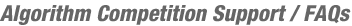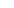JOINSample Problem Statements
Sample Problem StatementsHere are a couple of examples of the types of problems you can expect from a TopCoder competition. Once you become a TopCoder member, you can view all of the problems that TopCoder has ever used in the statistics section of our web site. If you're looking to practice, you'll find that after each TopCoder competition the problem sets that were used will be added as "practice rooms" in the TopCoder competition Arena. The TopCoder practice rooms are a great place to test your skills without the pressure of live competition and at the same time, get a feel for the competition environment. During a TopCoder competition, you'll find that the problem set for division two rooms is easier then the one for division one rooms. All first-time competitors will be placed in a division two competition room. Once your TopCoder rating reaches 1200, you'll be placed into the more difficult division one rooms. Below is an example of a problem statement from each of the two divisions. Example Division Two Problem Statement (From Single Round Match 96) A palindrome is a number that is the same whether it is read from left-to-right or right-to-left. For example, 121 and 34543 are both palindromes. It turns out that nearly every integer can be transformed into a palindrome by reversing its digits and adding it to the original number. If that does not create a palindrome, add the reverse of the new number to itself. A palindrome is created by repeating the process of reversing the number and adding it to itself until the number is a palindrome. Create a class Transform that contains the method palindrome, which takes a number N that is to be transformed and returns a number that is the resultant palindrome from this process. Of course if N is already a palindrome, return it without changing it. Though it is theorized that all numbers can be transformed to palindromes in this way, some numbers do not converge in a reasonable amount of time. For instance, 196 has been carried out to 26,000 digits without finding a palindrome. So if the method finds that the resultant palindrome must be greater than 1,000,000,000, return the special value -1 instead. DEFINITION Class: Transform Method: palindrome Parameters: int Returns: int Method signature (be sure your method is public): int palindrome(int N); NOTES - Leading zeroes are never considered part of a number when it is reversed. For instance, 12's reverse will always be 21 regardless of whether it is represented as 12, 012, or 0012. Examples with leading zeroes use the leading zeroes for clarity only. TopCoder will ensure the validity of the inputs. Inputs are valid if all of the following criteria are met: - N will be between 1 and 10000 inclusive. EXAMPLES Worked examples: Example 1: N = 28 28 + 82 = 110 110 + 011 = 121, a palindrome. Return 121 Example 2: N = 51 51 + 15 = 66, a palindrome. Return 66 Further examples: Example 3: N = 11, return 11 Example 4: N = 607, return 4444 Example 5: N = 196, return -1 Example Division One Problem Statement (From Single Round Match 102) Binary strings, as most of us know, are composed solely of 0's and 1's. Sometimes it is necessary to turn all the bits either on (1) or off (0). However, sometimes it is not possible to just pick and flip individual bits. In this hypothetical scenario, it is only possible to flip bits in a contiguous segment which is a subset of the contiguous segment which was flipped immediately prior to it. For example, if bits 2-4 are flipped, it is not legal to flip bits 3-5 next, or bits 1-3. However, bits 3-4 or bits 2-3 would be legal. The first segment to be flipped can be located anywhere in the sequence. Create a class BitFlipper which contains a method minFlip which determines the minimum number of bits that must be flipped under the above restriction in order to get all the bits set to 0. For purposes of this problem, to flip a bit means to change it from 0 to 1 or from 1 to 0. DEFINITION Class: BitFlipper Method: minFlip Parameters: String Returns: int Method signature (be sure it is declared public): int minFlip (String bits); TopCoder will ensure the validity of the inputs. Inputs are valid if all of the following criteria are met: * bits will be between 0 and 50 characters in length, inclusive * bits will contain only 1's and 0's. EXAMPLES Example 1: bits = "00110". By flipping bits 3-4, we get "00000". Method returns 2. Example 2: bits = "10110" If we flip bits 1-4, we get "01000". Now we flip bit 2 and get "00000". Method returns 4 + 1 = 5. Example 3: bits = "1001110001" Flipping bits 1-10 yields "0110001110" Now, flipping bits 2-9 yields "0001110000" Again, flipping bits 4-6 yields "0000000000" Method returns 10 + 8 + 3 = 21. Example 4: bits = "10001" Method returns 8. Example 5: bits = "101010101" Method returns 25. Example 6: bits = "" Method returns 0.